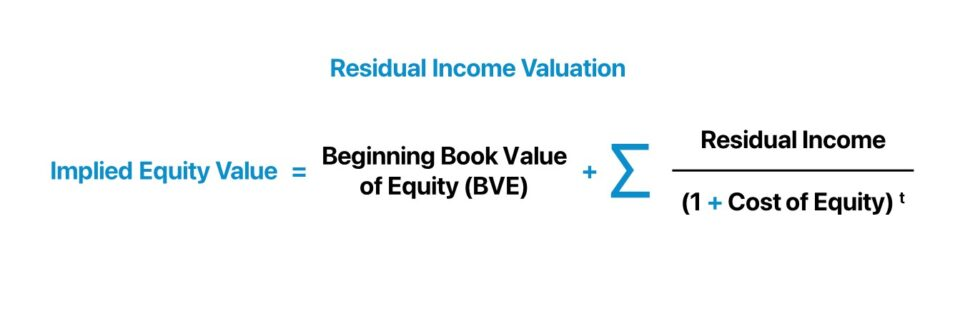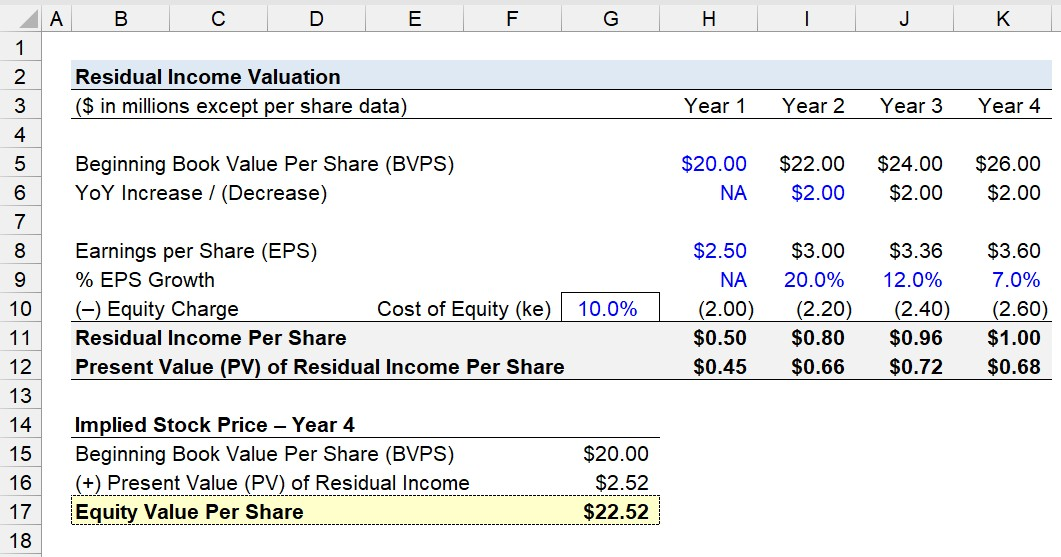Welcome to Wall Street Prep! Use code at checkout for 15% off.# Residual Income Valuation

Guide to Understanding the Residual Income Valuation Model## How to Calculate Residual Income Valuation

The residual income valuation model is an earnings-based method used to estimate the intrinsic value of equity of a given company.

Conceptually, the intrinsic value as calculated per the residual income model approach is the sum of two components:

1. Current Book Value of Equity (BVE)
2. Present Value (PV) of Projected Residual Income

The residual income, the discounted cash flow metric, is the profit of a company, adjusted by the cost of equity (ke).

The cost of equity (ke) is the minimum rate of return required by shareholders, which reflects the risk of investing in the equity issued by the underlying company.

The formula to calculate the residual income is as follows.

Residual Income = Net Profit  (Invested Capital × Cost of Equity)

The residual income can also be computed using the following equation.

Residual Income = Net Profit × (1  Cost of Equity ÷ Return on Investment)

## Residual Income Model vs. DCF Analysis: What is the Difference?

In practice, the residual income model is not as commonly used as the discounted cash flow (DCF) model for analyzing the valuation of a company.

The residual income model (RIM) is theoretically similar to the discounted cash flow (DCF) model and dividend discount model (DDM), since all three methods are oriented around forecasting the future performance of a company.

However, the residual income model is based on the current book value of equity (BVE), an accounting measure of profitability, and the future economic profits the company is expected to produce, which is adjusted by the cost of equity.

The residual income model notably assumes the company reinvests its earnings at the cost of equity (ke) and neglects other forms of capital, such as debt financing and preferred stock.

Unlike the DCF and DDM, the residual income model explicitly recognizes the costs of equity capital, i.e. the adjustment is termed the “equity charge”.

Equity Charge = Equity Capital × Cost of Equity (ke)

The limitation of the residual income model is that the approach is suited for companies that issue no dividends and consistent income.

## Residual Income Valuation Formula

The formula to calculate the equity value of a company under the residual income model is as follows.

Equity Value = Book Value of Equity (BVE) + Σ Residual Income ÷ (1 + r) ^ t

Where:

• Book Value of Equity (BVE) → The current book value of equity (BVE), or “Shareholders’ Equity”, as of the present date (t = 0).
• Σ Residual Income ÷ (1 + r) ^ t → The present value (PV) of residual income expected to be generated by the company, where the discount rate (r) is the cost of equity.

## Residual Income Valuation Calculator

We’ll now move to a modeling exercise, which you can access by filling out the form below.#### Excel TemplateSubmitting...

## Residual Income Valuation Calculation Example

Suppose we’re tasked with projecting the stock price of a publicly traded company four years from the present date using a short-form residual income valuation model.

Over the projection period – from Year 1 to Year 4 – the company’s book value of equity per share (BVPS) is initially \$20.00, which will increase by \$2.00 per annum to reach \$26.00 at the beginning of Year 4.

In the same time frame, the company’s earnings per share (EPS) starts at \$2.50 in Year 1, which will increase by a growth rate of 20.0%, 12.0%, and 7.0% for the remainder of the forecast period.

The equity charge per period is equal to the beginning BVPS multiplied by the cost of equity, which we’ll assume is 10.0%.

The residual income per share can be determined by subtracting the corresponding equity charge from the earnings per share (EPS) figure in each period.

Once completed, we must discount the residual income per share back to the present date using the cost of equity (ke).

For example, the present value (PV) of the residual income per share in Year 4 is \$0.68.

• Present Value (PV) of Residual Income Per Share = \$1.00 ÷ (1 + 10%)^4 = \$0.68

In the final step, we’ll add the initial beginning book value of equity per share (BVPS) to the sum of the present value (PV) of the company’s residual income.

In conclusion, the implied equity value per share (or stock price) derived from the residual income valuation model is \$22.52 at the start of Year 4.

• Implied Equity Value Per Share = \$22.52Step-by-Step Online Course

### Learn Buy-Side (Hedge Fund) Modeling

Led by a former hedge fund PM (Maverick, Citadel, DE Shaw, Schonfeld), this program begins where financial modeling training ends — with a deep-dive into how buy-side analysts build financial models to make key investment decisions.